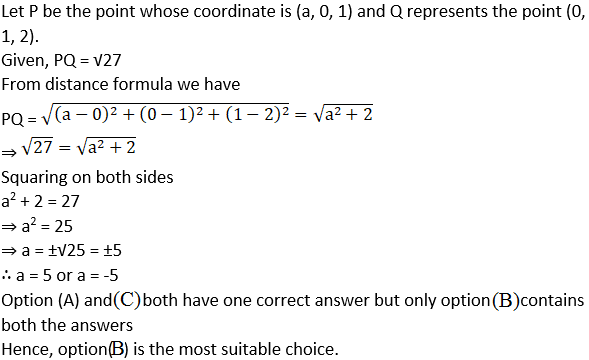# NCERT Exemplar Solutions for Class 11 Maths Chapter 12 Introduction to Three Dimensional Geometry

NCERT Exemplar Solutions for Class 11 Maths Chapter 12 Introduction to Three Dimensional Geometry are prepared after vast research by highly experienced Maths teachers at BYJU’S. The NCERT Exemplar is the best book available, based on the CBSE syllabus. The description provided in the PDFs enhances conceptual understanding among the students, which in turn, helps in solving problems without mistakes. The solutions are free to download, and the questions are arranged systematically to make preparation and solving different types of questions easy. In order to score good marks, students are advised to learn these NCERT Exemplar Solutions.

The 12th Chapter of NCERT Exemplar Solutions for Class 11 Introduction to Three Dimensional Geometry explains the distance formula and section formula. By practising these solutions, students can score high in their exams. Some of the essential topics of this chapter are listed below.

• Coordinate axes and coordinate planes
• Coordinate of a point in space
• Sign of coordinates of a point
• Distance formula
• Section formula

## Download the PDF of NCERT Exemplar Solutions for Class 11 Maths Chapter 12 Introduction to Three dimensional Geometry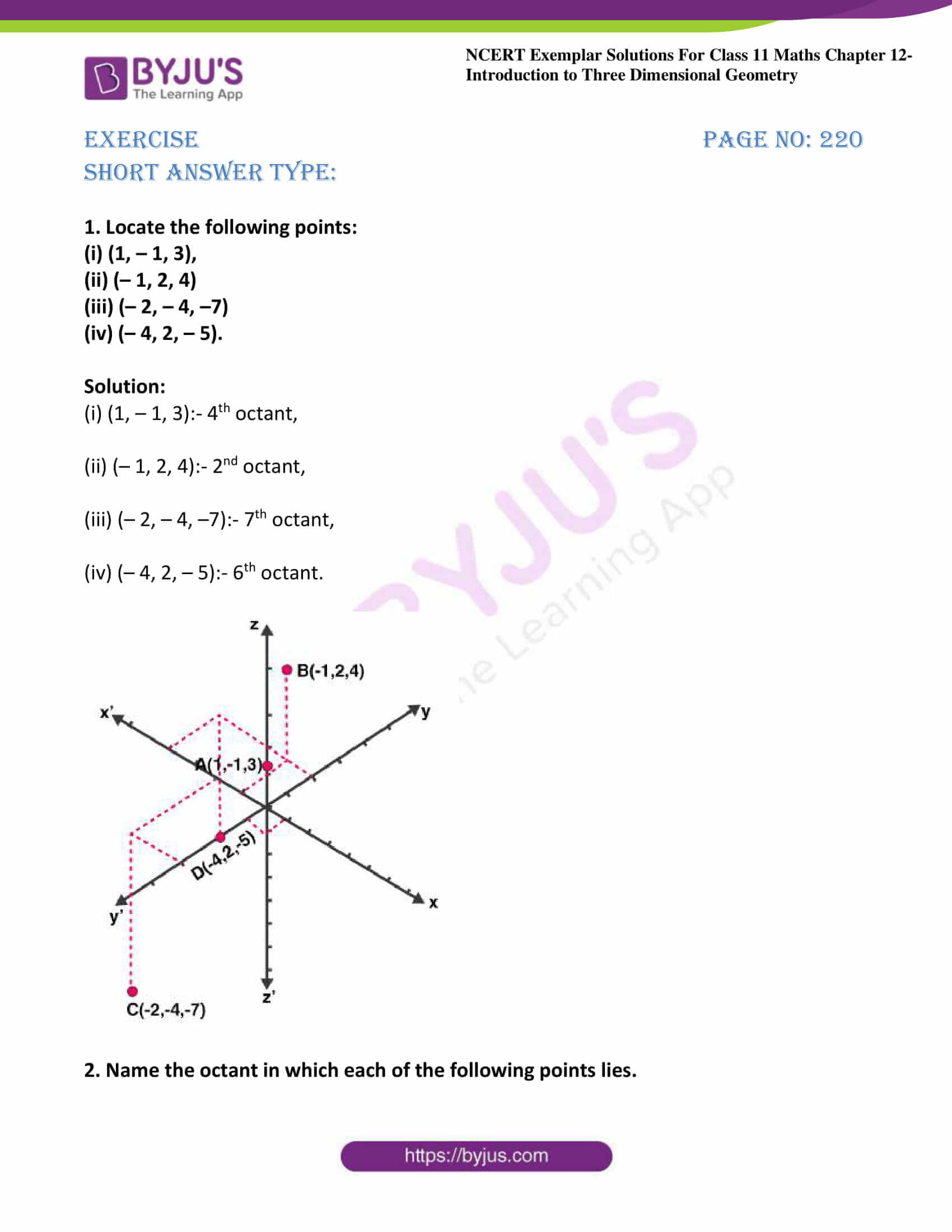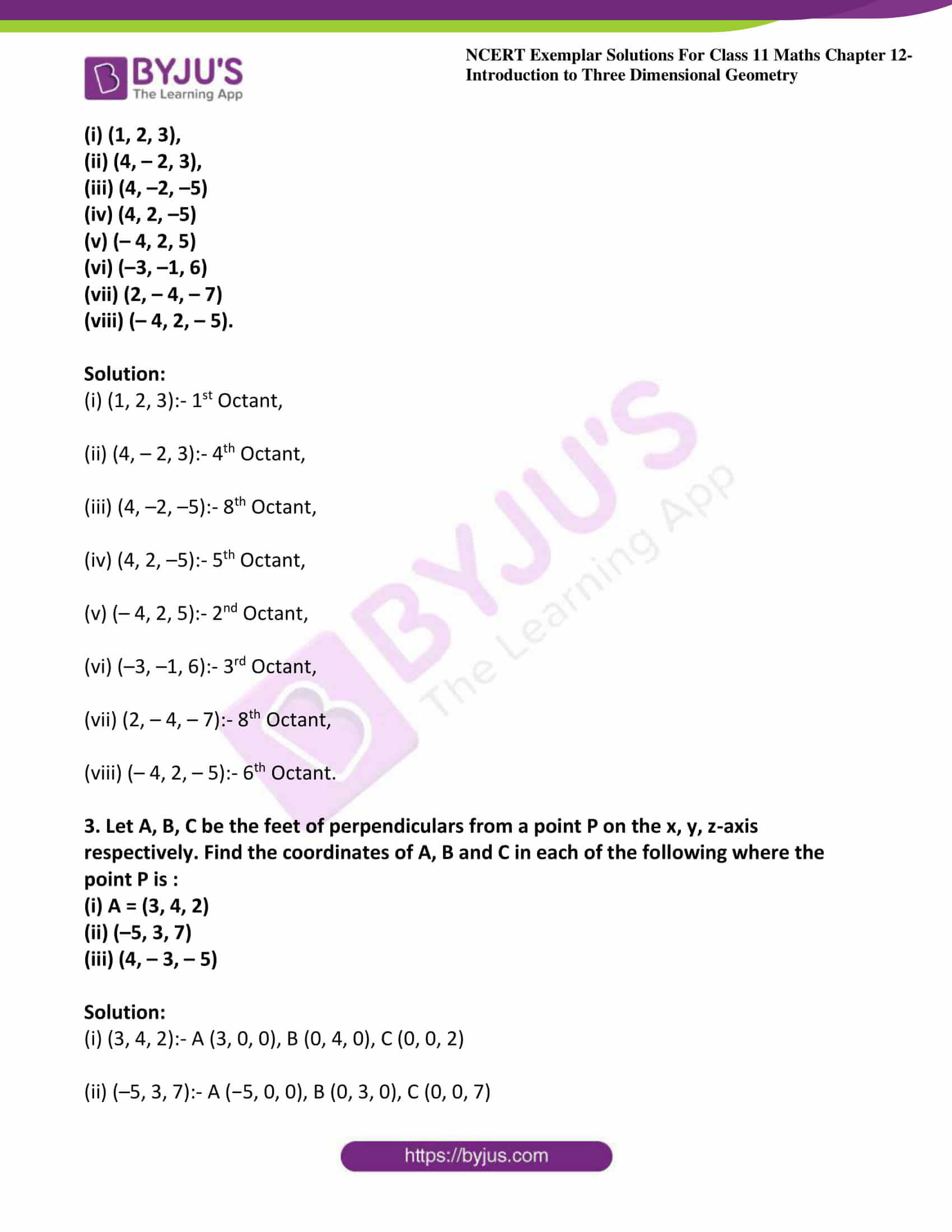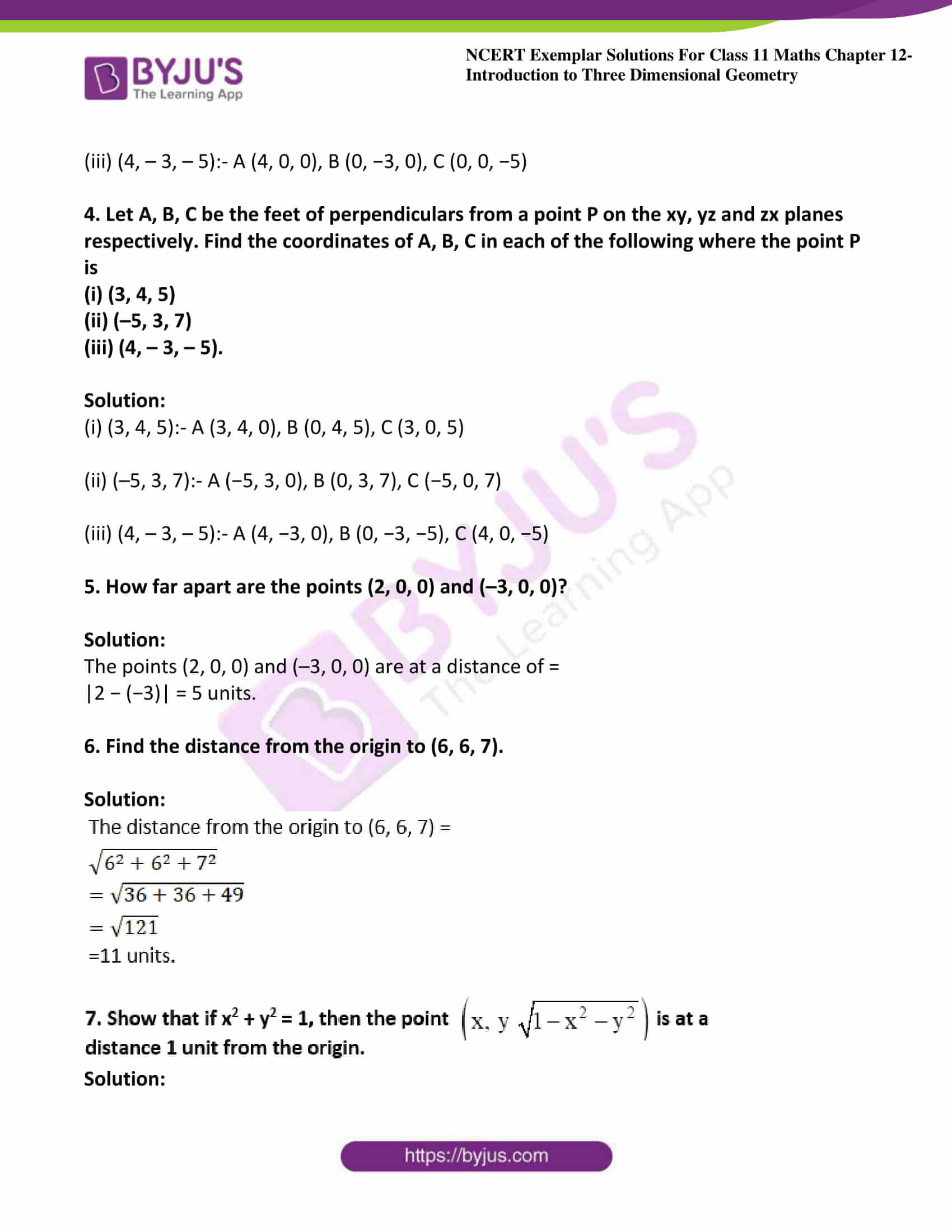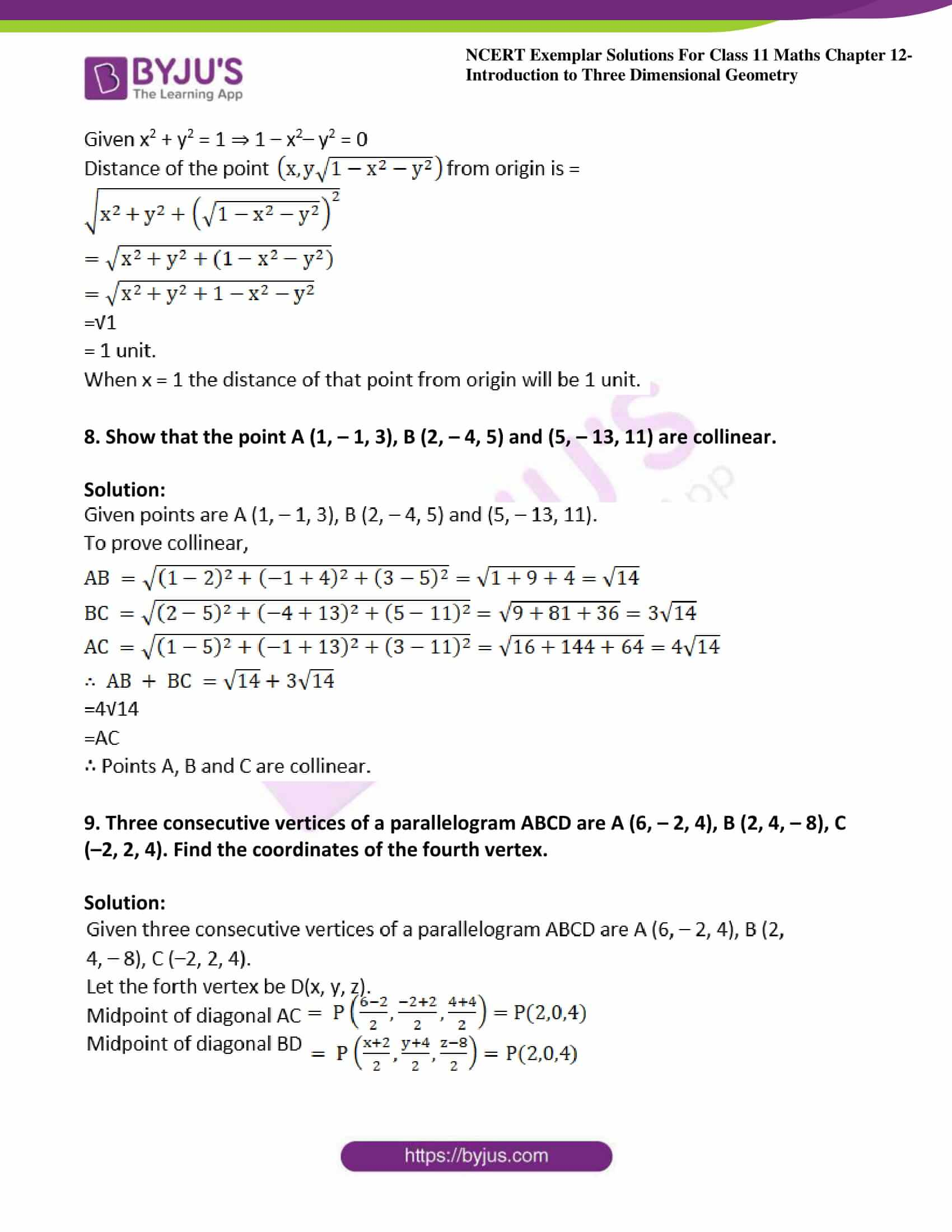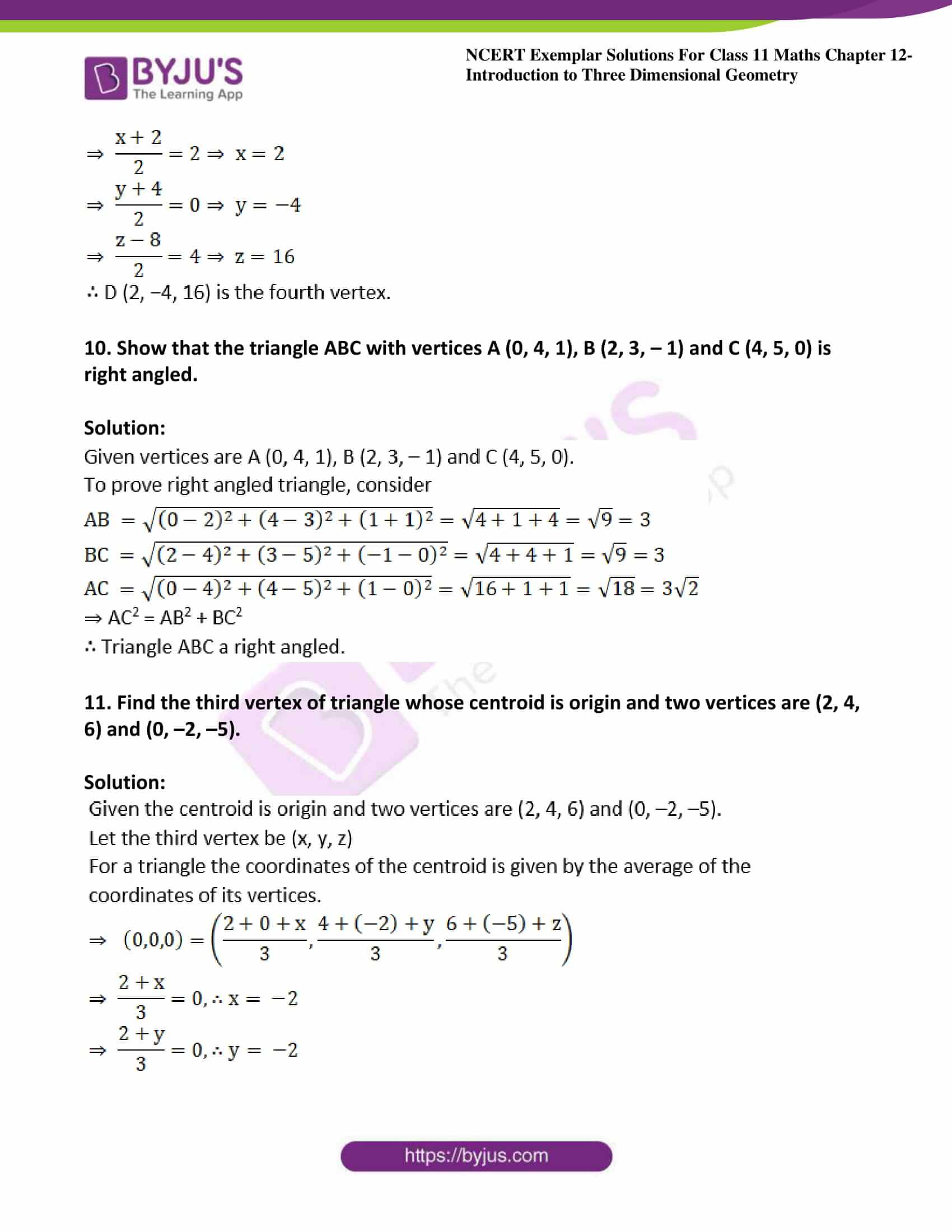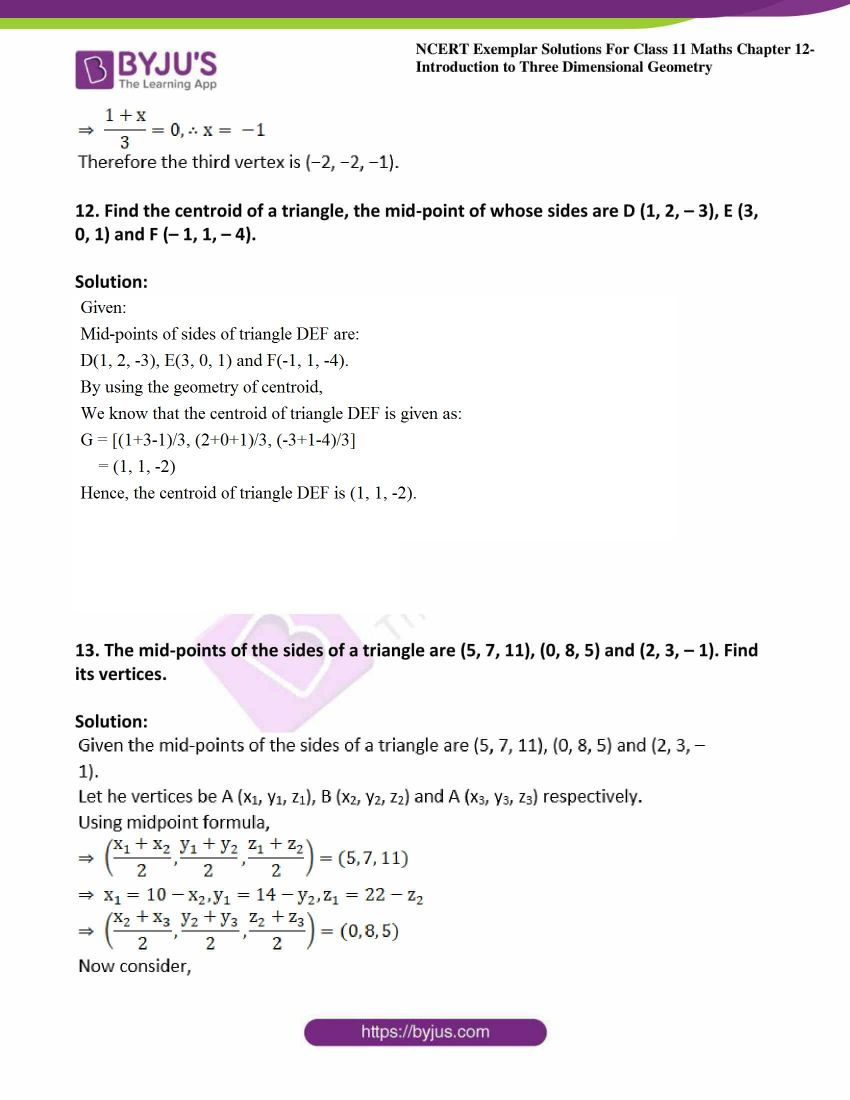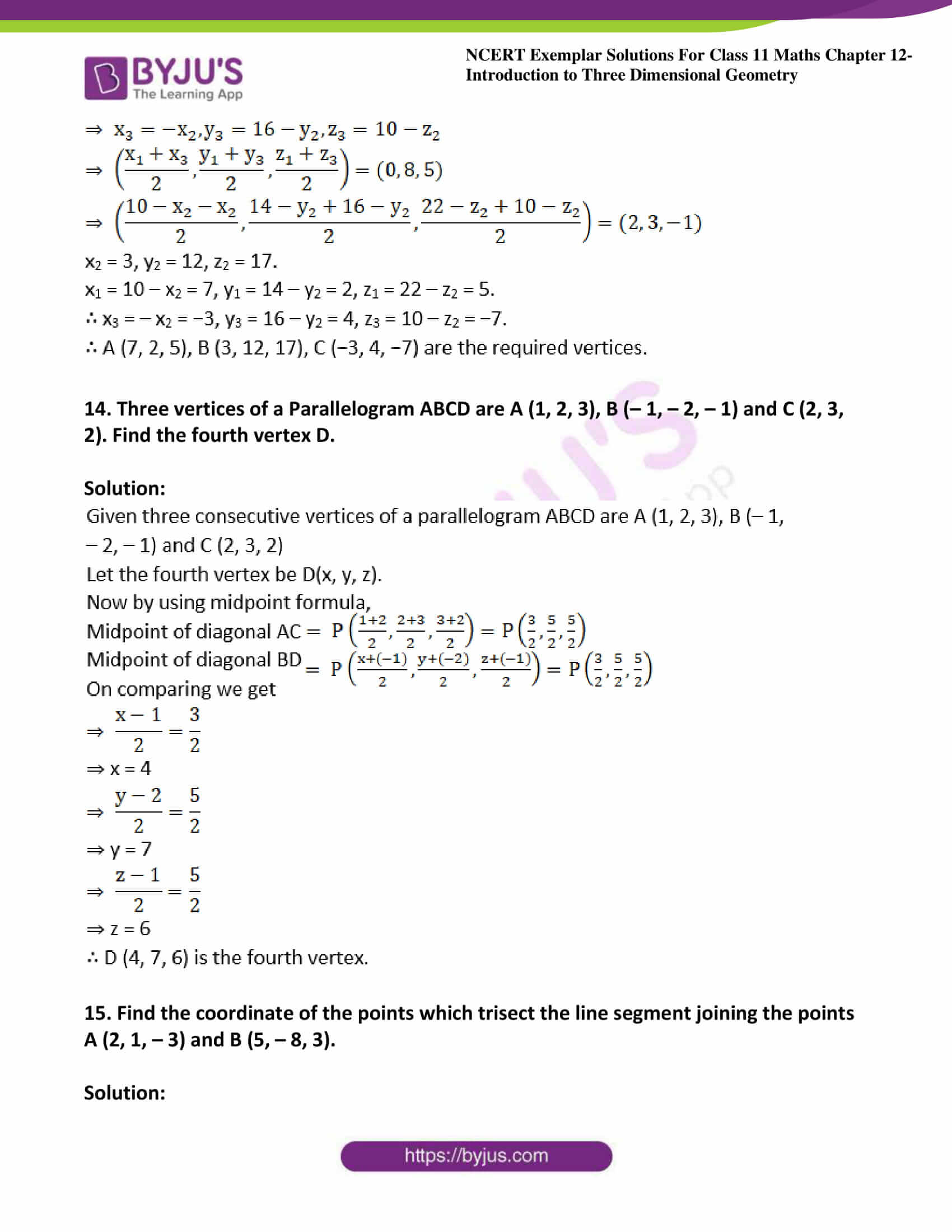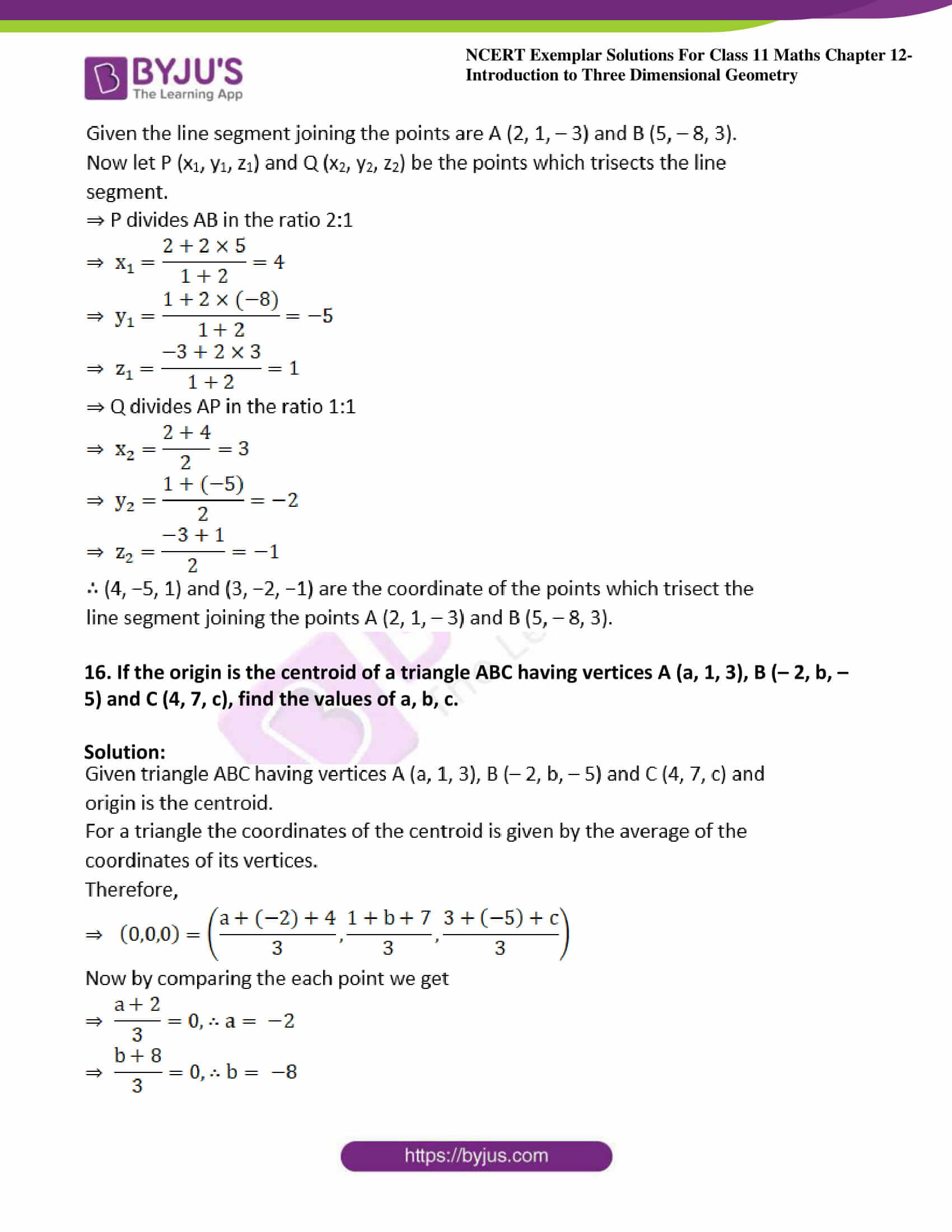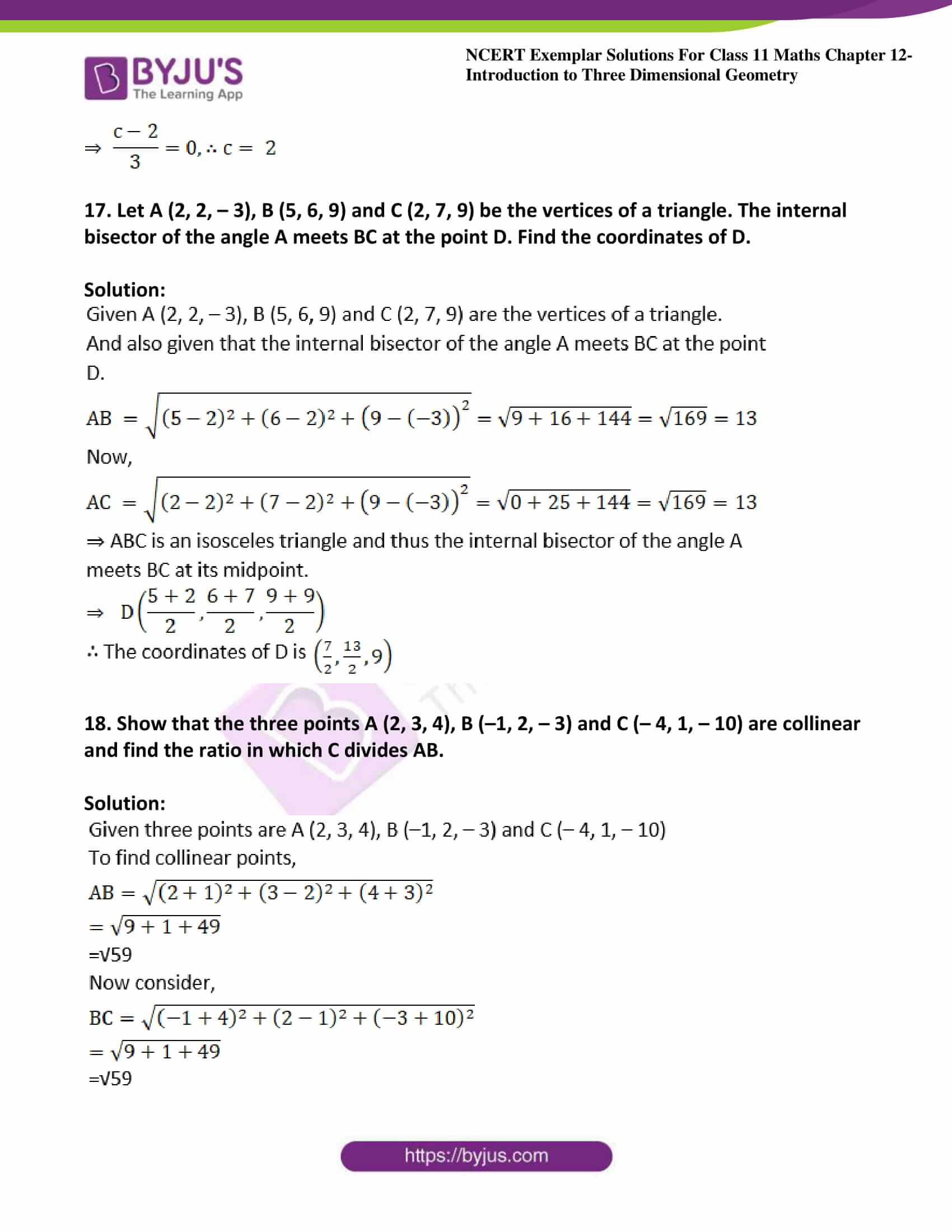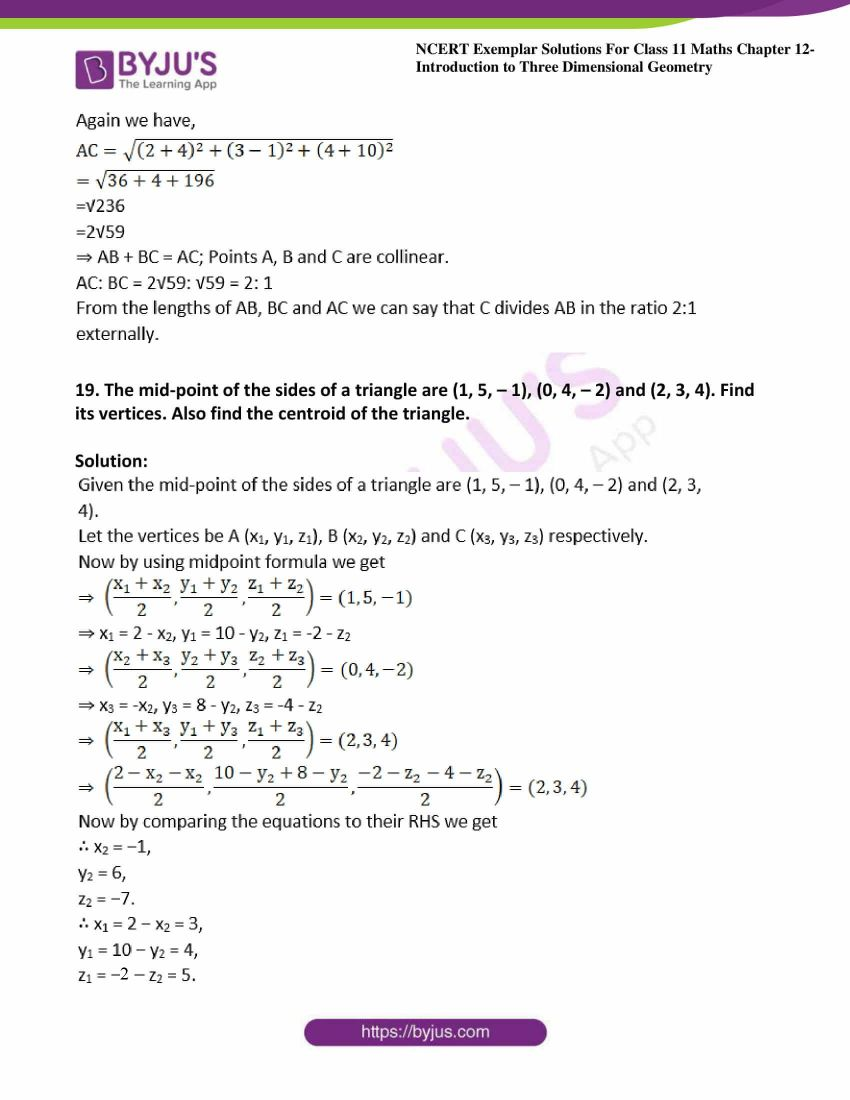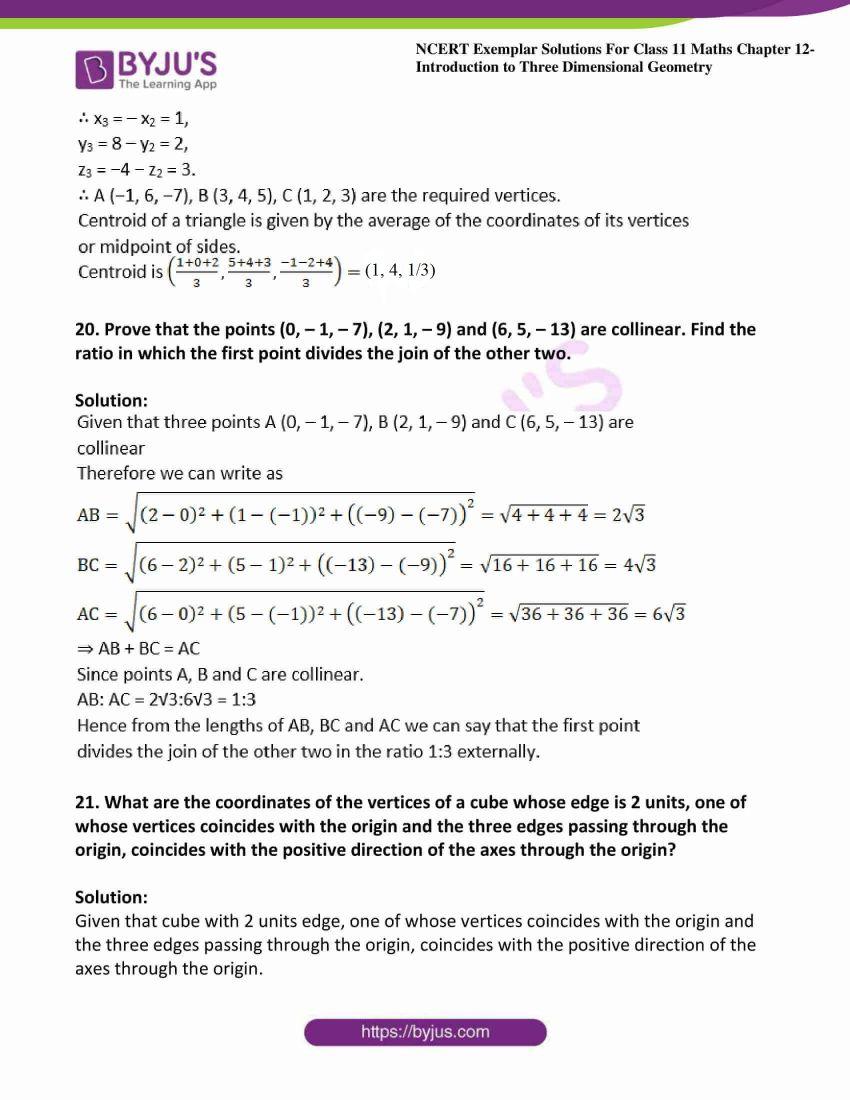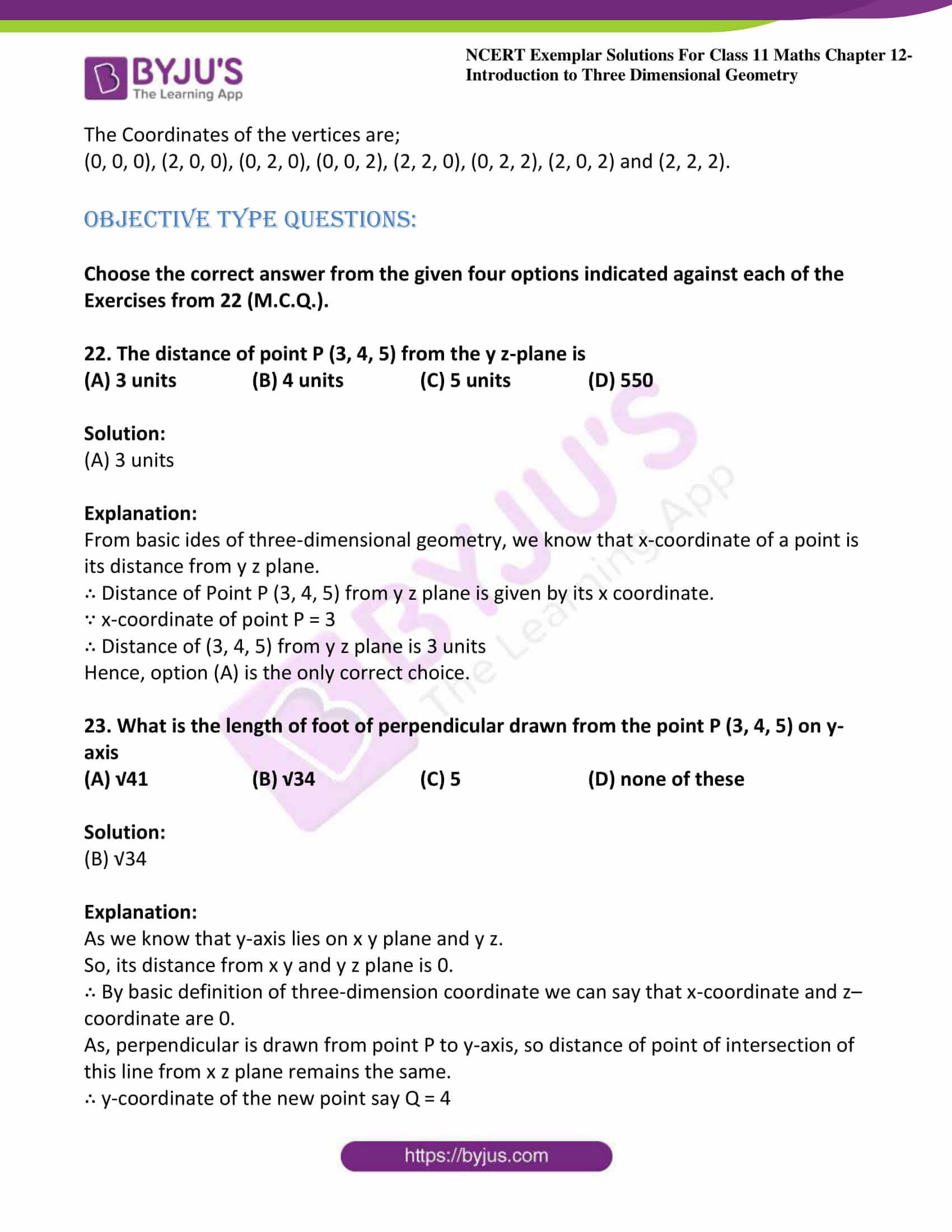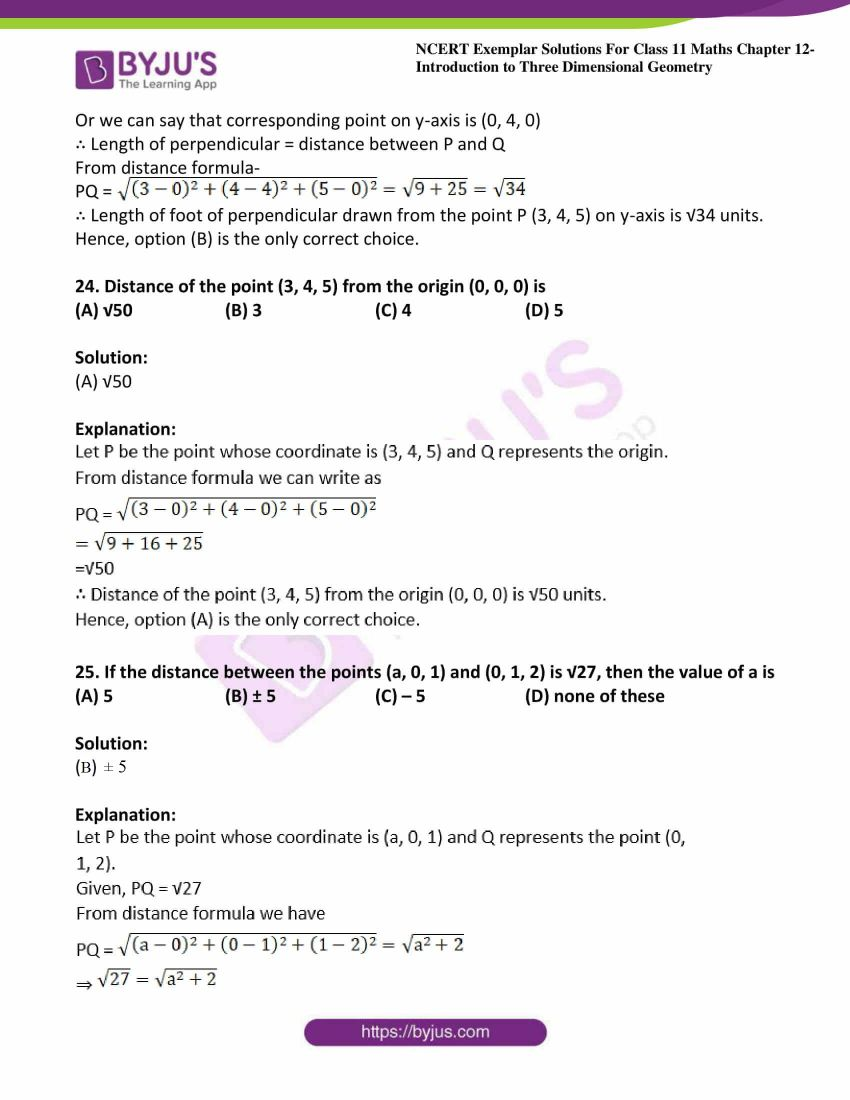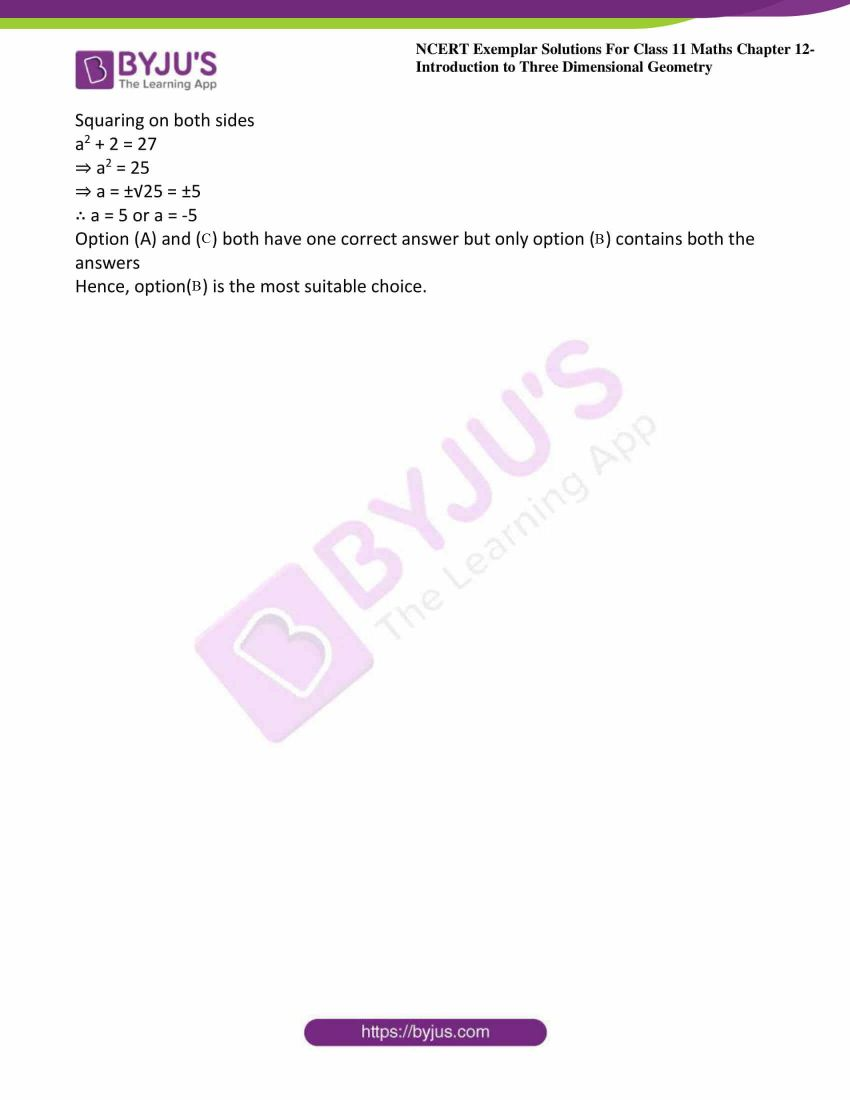### Access Answers to NCERT Exemplar Solutions for Class 11 Maths Chapter 12 – Introduction to Three Dimensional Geometry

Exercise Page No: 220

1. Locate the following points:
(i) (1, – 1, 3),

(ii) (– 1, 2, 4)
(iii) (– 2, – 4, –7)

(iv) (– 4, 2, – 5).

Solution:

(i) (1, – 1, 3):- 4th octant,

(ii) (– 1, 2, 4):- 2nd octant,

(iii) (– 2, – 4, –7):- 7th octant,

(iv) (– 4, 2, – 5):- 6th octant.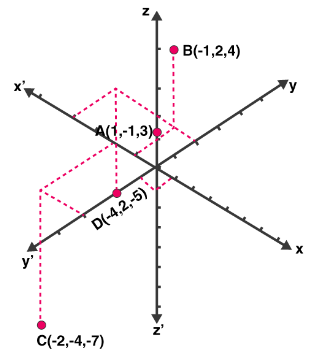2. Name the octant in which each of the following points lies.

(i) (1, 2, 3),

(ii) (4, – 2, 3),

(iii) (4, –2, –5)

(iv) (4, 2, –5)
(v) (– 4, 2, 5)

(vi) (–3, –1, 6)

(vii) (2, – 4, – 7)

(viii) (– 4, 2, – 5).

Solution:

(i) (1, 2, 3):- 1st Octant,

(ii) (4, – 2, 3):- 4th Octant,

(iii) (4, –2, –5):- 8th Octant,

(iv) (4, 2, –5):- 5th Octant,

(v) (– 4, 2, 5):- 2nd Octant,

(vi) (–3, –1, 6):- 3rd Octant,

(vii) (2, – 4, – 7):- 8th Octant,

(viii) (– 4, 2, – 5):- 6th Octant.

3. Let A, B, C be the feet of perpendiculars from a point P on the x, y, z-axis respectively. Find the coordinates of A, B and C in each of the following where the point P is :
(i) A = (3, 4, 2)

(ii) (–5, 3, 7)

(iii) (4, – 3, – 5)

Solution:

(i) (3, 4, 2):- A (3, 0, 0), B (0, 4, 0), C (0, 0, 2)

(ii) (–5, 3, 7):- A (−5, 0, 0), B (0, 3, 0), C (0, 0, 7)

(iii) (4, – 3, – 5):- A (4, 0, 0), B (0, −3, 0), C (0, 0, −5)

4. Let A, B, C be the feet of perpendiculars from a point P on the xy, yz and zx planes respectively. Find the coordinates of A, B, C in each of the following where the point P is
(i) (3, 4, 5)

(ii) (–5, 3, 7)

(iii) (4, – 3, – 5).

Solution:

(i) (3, 4, 5):- A (3, 4, 0), B (0, 4, 5), C (3, 0, 5)

(ii) (–5, 3, 7):- A (−5, 3, 0), B (0, 3, 7), C (−5, 0, 7)

(iii) (4, – 3, – 5):- A (4, −3, 0), B (0, −3, −5), C (4, 0, −5)

5. How far apart are the points (2, 0, 0) and (–3, 0, 0)?

Solution:

The points (2, 0, 0) and (–3, 0, 0) are at a distance of =

|2 − (−3)| = 5 units.

6. Find the distance from the origin to (6, 6, 7).

Solution: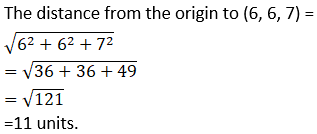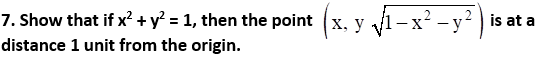Solution: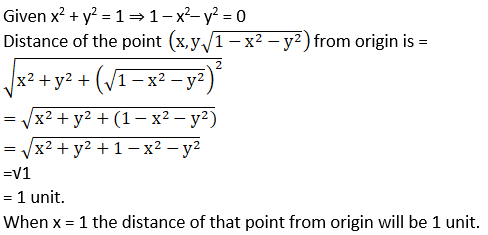8. Show that the point A (1, – 1, 3), B (2, – 4, 5) and (5, – 13, 11) are collinear.

Solution: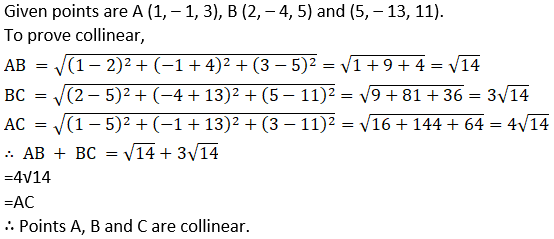9. Three consecutive vertices of a parallelogram ABCD are A (6, – 2, 4), B (2, 4, – 8), C (–2, 2, 4). Find the coordinates of the fourth vertex.

Solution: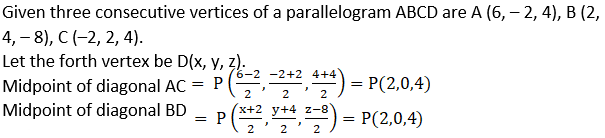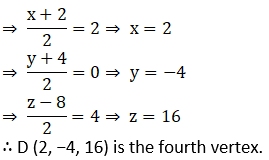10. Show that the triangle ABC with vertices A (0, 4, 1), B (2, 3, – 1) and C (4, 5, 0) is right angled.

Solution: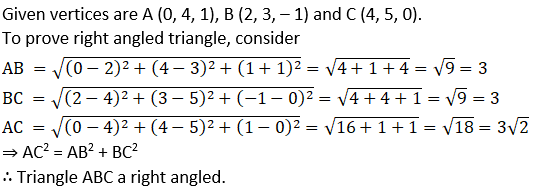11. Find the third vertex of triangle whose centroid is origin and two vertices are (2, 4, 6) and (0, –2, –5).

Solution: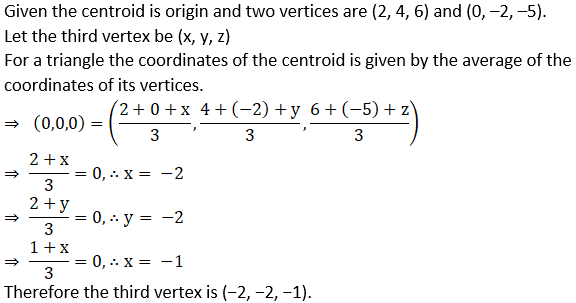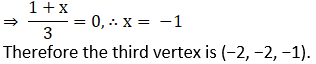12. Find the centroid of a triangle, the mid-point of whose sides are D (1, 2, – 3), E (3, 0, 1) and F (– 1, 1, – 4).

Solution: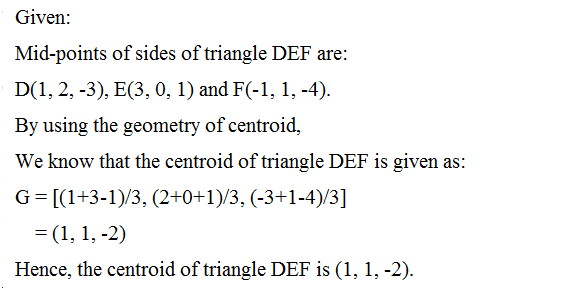13. The mid-points of the sides of a triangle are (5, 7, 11), (0, 8, 5) and (2, 3, – 1). Find its vertices.

Solution: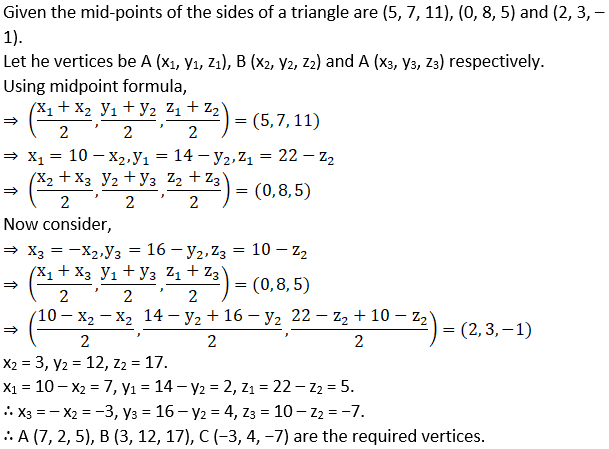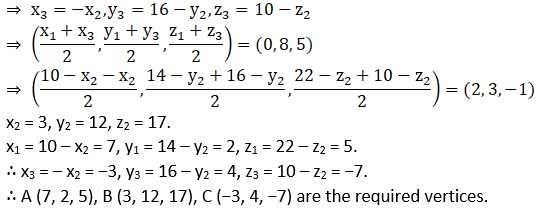14. Three vertices of a Parallelogram ABCD are A (1, 2, 3), B (– 1, – 2, – 1) and C (2, 3, 2). Find the fourth vertex D.

Solution: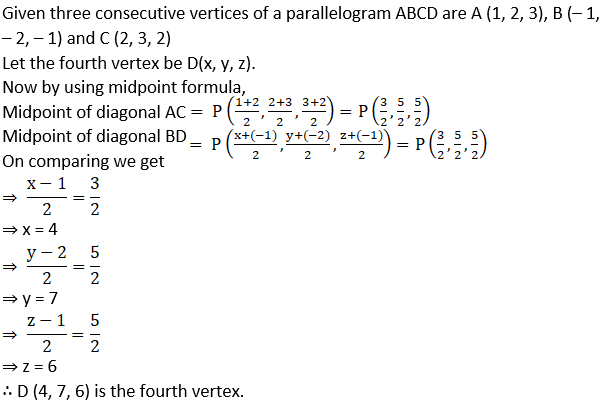15. Find the coordinate of the points which trisect the line segment joining the points A (2, 1, – 3) and B (5, – 8, 3).

Solution: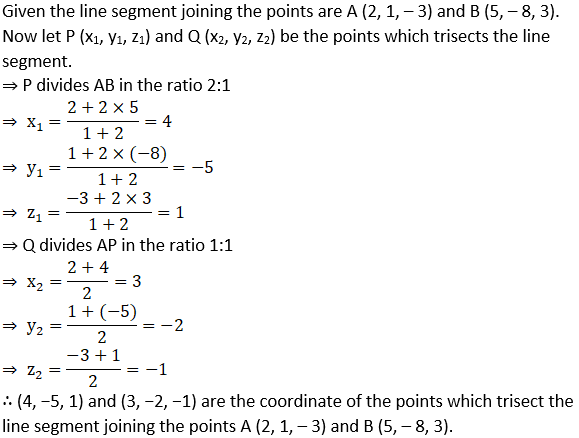16. If the origin is the centroid of a triangle ABC having vertices A (a, 1, 3), B (– 2, b, – 5) and C (4, 7, c), find the values of a, b, c.

Solution: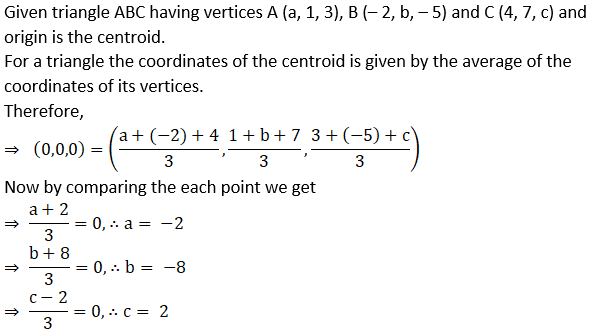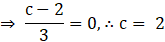17. Let A (2, 2, – 3), B (5, 6, 9) and C (2, 7, 9) be the vertices of a triangle. The internal bisector of the angle A meets BC at the point D. Find the coordinates of D.

Solution: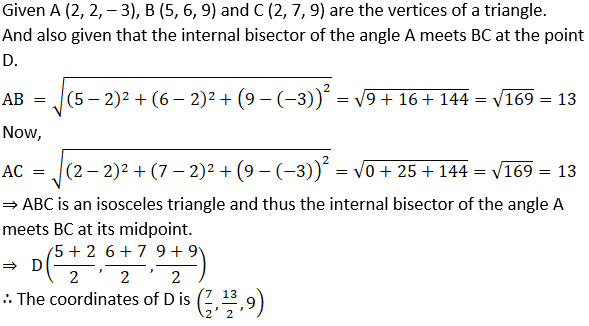18. Show that the three points A (2, 3, 4), B (–1, 2, – 3) and C (– 4, 1, – 10) are collinear and find the ratio in which C divides AB.

Solution: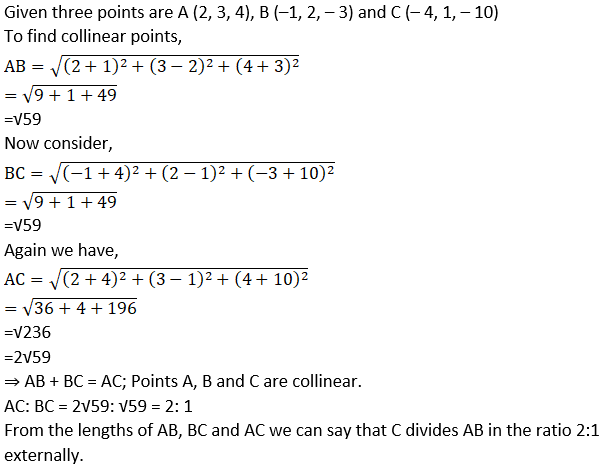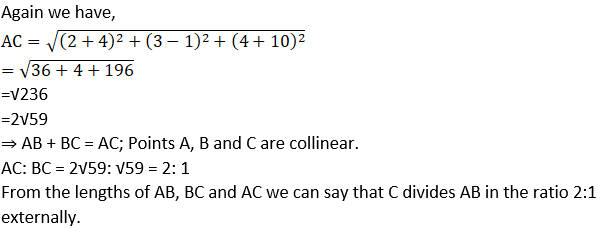19. The mid-point of the sides of a triangle are (1, 5, – 1), (0, 4, – 2) and (2, 3, 4). Find its vertices. Also find the centroid of the triangle.

Solution: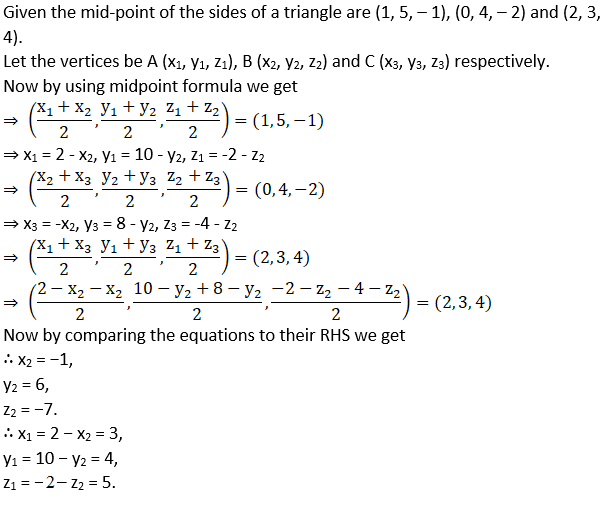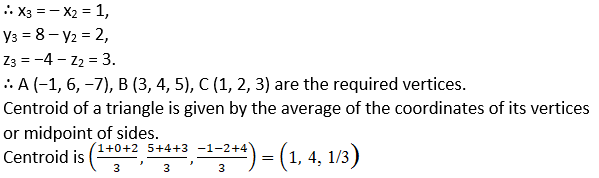20. Prove that the points (0, – 1, – 7), (2, 1, – 9) and (6, 5, – 13) are collinear. Find the ratio in which the first point divides the join of the other two.

Solution: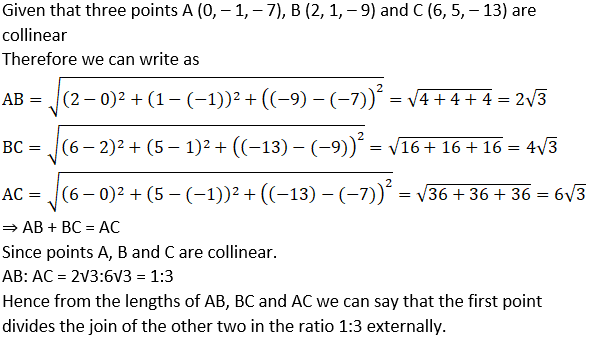21. What are the coordinates of the vertices of a cube whose edge is 2 units, one of whose vertices coincides with the origin and the three edges passing through the origin, coincides with the positive direction of the axes through the origin?

Solution:

Given that cube with 2 units edge, one of whose vertices coincides with the origin and the three edges passing through the origin, coincides with the positive direction of the axes through the origin.

The Coordinates of the vertices are;

(0, 0, 0), (2, 0, 0), (0, 2, 0), (0, 0, 2), (2, 2, 0), (0, 2, 2), (2, 0, 2) and (2, 2, 2).

Objective type Questions:

Choose the correct answer from the given four options indicated against each of the Exercises from 22 (M.C.Q.).

22. The distance of point P (3, 4, 5) from the y z-plane is

(A) 3 units (B) 4 units (C) 5 units (D) 550

Solution:

(A) 3 units

Explanation:

From basic ides of three-dimensional geometry, we know that x-coordinate of a point is its distance from y z plane.

∴ Distance of Point P (3, 4, 5) from y z plane is given by its x coordinate.

∵ x-coordinate of point P = 3

∴ Distance of (3, 4, 5) from y z plane is 3 units

Hence, option (A) is the only correct choice.

23. What is the length of foot of perpendicular drawn from the point P (3, 4, 5) on y-axis

(A) √41 (B) √34 (C) 5 (D) none of these

Solution:

(B) √34

Explanation:

As we know that y-axis lies on x y plane and y z.

So, its distance from x y and y z plane is 0.

∴ By basic definition of three-dimension coordinate we can say that x-coordinate and z–coordinate are 0.

As, perpendicular is drawn from point P to y-axis, so distance of point of intersection of this line from x z plane remains the same.

∴ y-coordinate of the new point say Q = 4

Or we can say that corresponding point on y-axis is (0, 4, 0)

∴ Length of perpendicular = distance between P and Q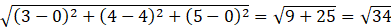From distance formula-

PQ =

∴ Length of foot of perpendicular drawn from the point P (3, 4, 5) on y-axis is √34 units.

Hence, option (B) is the only correct choice.

24. Distance of the point (3, 4, 5) from the origin (0, 0, 0) is

(A) √50 (B) 3 (C) 4 (D) 5

Solution:

(A) √50

Explanation: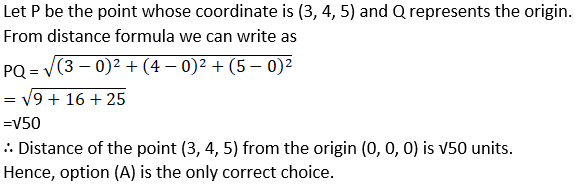25. If the distance between the points (a, 0, 1) and (0, 1, 2) is √27, then the value of a is (A) 5 (B) ± 5 (C) – 5 (D) none of these

Solution:

(B) ± 5

Explanation: